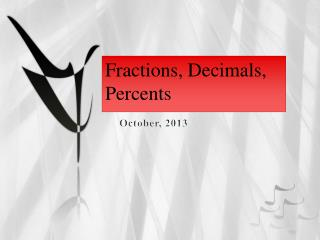DownloadDownload PresentationFractions, Decimals, Percents

# Fractions, Decimals, Percents

Télécharger la présentation## Fractions, Decimals, Percents

- - - - - - - - - - - - - - - - - - - - - - - - - - - E N D - - - - - - - - - - - - - - - - - - - - - - - - - - -
##### Presentation Transcript

1. Fractions, Decimals, Percents October, 2013

2. Reminder… • Numerator represents the… “part” • Denominator represents the… “whole” • So, • Example: There were 15 elephants in the zoo that has 236 animals. Write a fraction representing this situation.

3. Types of Decimals • Examine the decimal below. What do you notice? • 6.375 • Terminating Decimal: Any decimal that “terminates” • “Terminates” means “to end or stop” • Examples of Terminating Decimals: • 5.67 4.23 • -6.901 -3.75 • A terminating decimal is part of the real number system. How is it classified?

4. Examine the decimals below. What do you notice? • 6.33333…. • Repeating Decimal: Any decimal that has a constant pattern of digits that repeat. • Examples: • 5.3333… “3” is the repeating digit • 6.3030… “30” is the repeating pattern • -1.233233… “233” is the repeating pattern

5. Repeating Decimals • A repeating decimal is part of the real number system. How is it classified? • Bar Notation: • The line above the repeating digits • only occurs over the repeating part • Examples: • 1.34 1.23 16.598

6. Fractions, Decimals & Percents • All three have the same value, they are just represented in different ways. • CANcalculate with fractions AND decimals! • CANNOT calculate with percents!

7. Note to self: • Use “Examples” document for practice on each section.

8. FRACTIONS TO DECIMALS

9. Fractions to Decimals • Any fraction can be written as a decimal – • How? • Divide the numerator by the denominator. • YOU MUST BE ABLE TO SHOW THIS USING LONG DIVISION!! 5/8 = .625 2/5 = .40

10. FRACTIONS TO PERCENTS

11. Fractions to Percents • Percent means… • “Per 100” or “Out of 100”

12. Fractions to Percents • Make the fraction a decimal and multiply by 100 • (move decimal 2 places to right) • 1/4 = .25 = 25% • 12/25 = .48 = 48%

13. You try! • Fraction to Decimal: (use long division) • Fraction to Percent:

14. DECIMALS TO FRACTIONS

15. Decimals to Fractions • “SAY” the decimal and create the fraction …then, simplify! • .35 = 35/100 = 7/20 • .217 = 217/1000

16. Repeating Decimals to Fractions • How do you make a repeating decimal into a fraction? • There is a very complicated process that ultimately says “take the repeating part of the decimal and put it over a denominator that has the same number of 9’s.” • Example – 0.3333… = 3/9 = 1/3 .484848… = 48/99

17. DECIMALS TO PERCENTS

18. Decimals to Percents • Multiply by 100 (move decimal 2 places to right) • .25 = 25% • .48 = 48%

19. Decimals to Percents • D ----- P • D ------- P

20. You Try! • Decimals to Fractions: • 0.52 0.8 • Decimals to Percents: • 0.13 0.192

21. PERCENTS TO FRACTIONS

22. Percents to Fractions • Percent = “Per 100” or “Out of 100”… • Removing the % sign and placing the number over 100. • 23% = 23/100 • 55% = 55/100 (simplify)

23. PERCENTS TO DECIMALS

24. Percents to Decimals • Divide the percent by 100 (move decimal 2 places to the left) • 23% = .23 • 64% = .64

25. Percents to Decimals • D ----- P • D ------- P

26. You Try! • Percents to Fractions: • 85% 1% • Percents to Decimals: • 21% 27.3%

27. Quick Tip – Decimal/Percent • .83 • 46%

28. FormativeClean Sheet – Practice – 1 of 3 • Write each fraction as a decimal (use long division). • 1/8 5/9 7 ½ • Write each decimal as a fraction. • .22 0.1 4.6

29. Practice – 2 of 3 • Write each fraction as a percent. • ¼ 6/10 17/20 • Write each percent as a fraction. • 90% 75% 22%

30. Practice – 3 of 3 • Write each percent as a decimal. • 27% 70% 6% • Write each decimal as a percent. • .95 .08 5.8